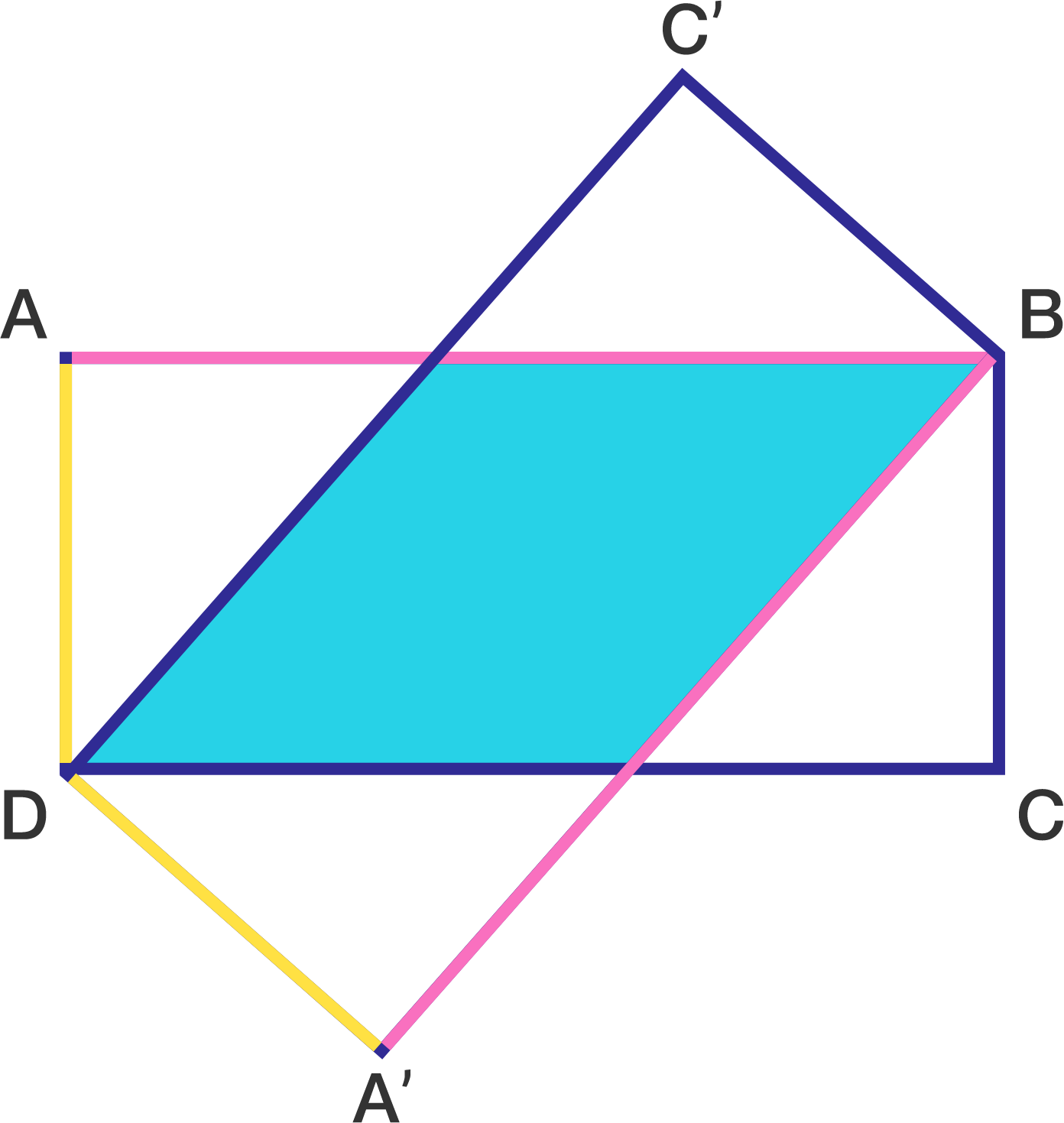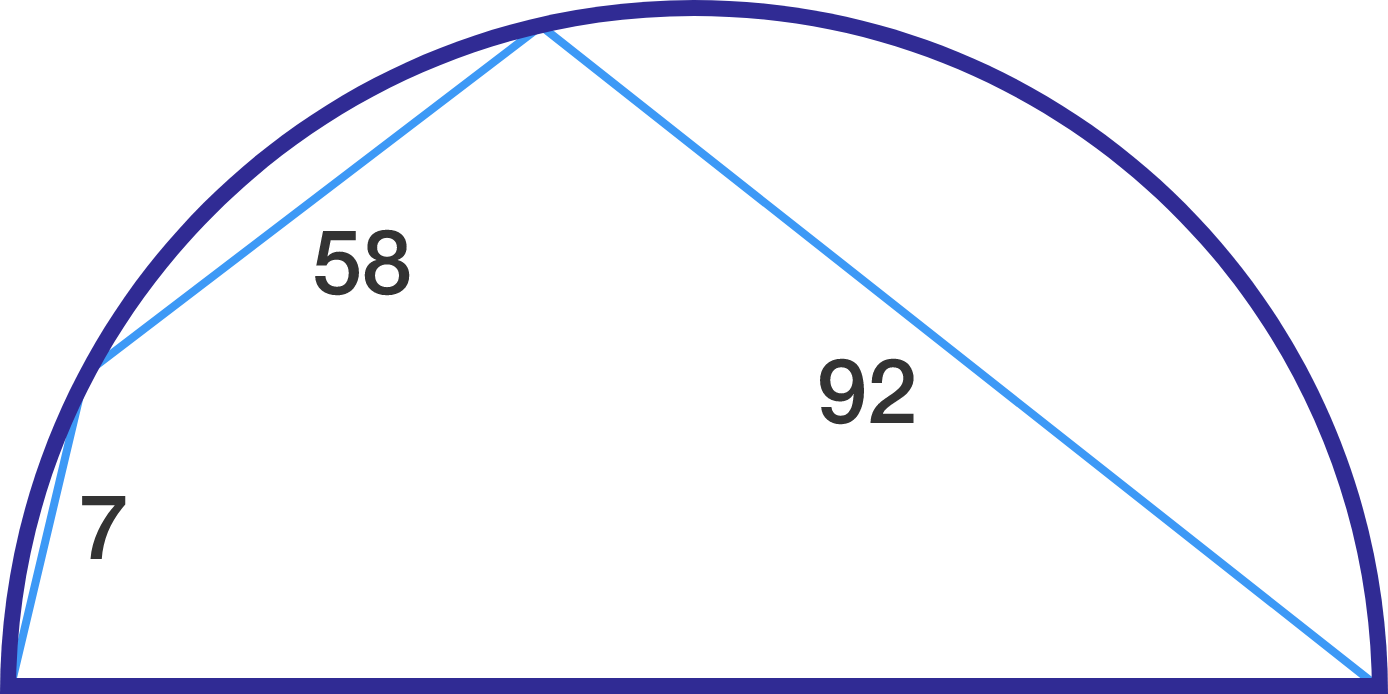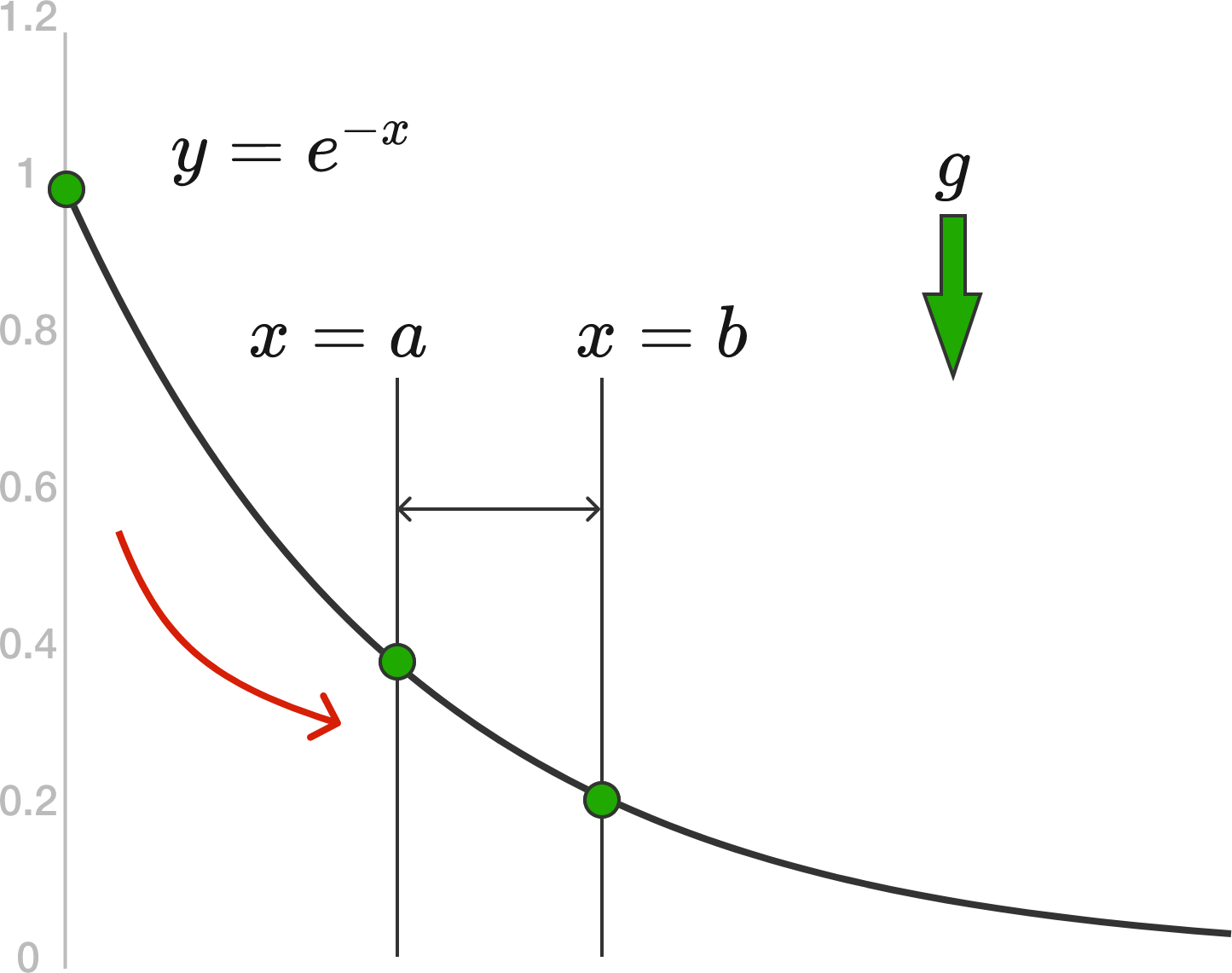# Problems of the Week

Contribute a problem

# 2017-04-24 Intermediate

The last digit of 2 is 2.

The last digit of $2^2$ is $2+2$.

The last digit of $2^{2^2}$ is $2+2+2$.

The last digit of $2^{2^{2^2}}$ is $\text{\_\_\_\_\_\_\_\_\_} .$$ABCD$ and $A'BC'D$ are both rectangles with side lengths $AD = A'D = 2,\quad AB =A'B = 6.$ Find the area of shaded region (to 2 decimal places).

The following is a conversation between Gabriel and Heather:

Gabriel: "I am thinking of two distinct single-digit numbers. Can you guess the sum of these two numbers?"
Heather: "No. Can you give me a clue?"

Gabriel: "The last digit of the product of the two numbers is your house number."
Heather: "Now I know the sum of the two numbers."

So, what is the sum of the two numbers?

Note: It is possible that the product of the two numbers is a single-digit.

What is the diameter of the semicircle in the diagram below?Note: The diagram is not drawn to scale.A bead slides under the pull of gravity, $g,$ down a frictionless wire segment in the shape of the curve $y = e^{-x}$, where $x$ is the horizontal direction and $y$ is the vertical direction. The bead starts from rest at $(x,y) = (0,1)$.

The time it takes for the particle to travel between $x=a$ and $x=b$ can be expressed as

$\large{t_{a,b} = \frac{1}{\sqrt{2g}} \int_a^b \sqrt{\frac{1 + P e^{Q x}}{1 + R e^{S x} }}\,dx},$

where $P,Q,R,$ and $S$ are integers.

Determine $P + Q + R + S.$

Note: The constant $e$ is Euler's number.

×